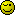# Why does this PDO->beginTransaction() eventually work OK

I followed the manual, tutorials, etc and EVENTUALLY managed to find the problem but still cannot understand why the following script is working:

``````  \$conn->beginTransaction(); // \$conn->commit();
\$stmt = \$conn->prepare
(
"
INSERT INTO `booksDasa`
( `ref`, `author`, `memo`, `type`, `baht`, `qqq` )
VALUES
( ?,?,?,?,?,? )
"
);

\$imax = 0;
foreach(\$aInserts as \$id => \$aFails):
\$aWorks = [];
foreach(\$aFails as \$id2 => \$tmp):
\$aWorks[] = \$tmp;
endforeach;

// SHOW FIRST ITEM ONLY
if(\$id):
// DO NOTHING
else:
prettyShow(\$aFails, '\$aFails');
prettyShow(\$aWorks, '\$aWorks');
endif;
\$ok = \$stmt->execute( \$aWorks );

\$imax++;
if(\$imax >= 2): break; endif;
endforeach;

\$ok = \$conn->commit();

``````

### Output

Edit:
In case you didn’t notice the passed \$aWorks array has numeric array keys whereas the aFailed array uses named keys.
I would have thought the passed data was the same. Is this a bug?

Well aWorks is definitely numeric:
`\$aWorks[] = \$tmp;`
No key = next numeric key.

I… dont see where \$aInserts is being defined though. \$aFails is defined by: `\$aInserts as \$id => \$aFails`
so \$aFails will have the same structure as the elements of \$aInserts.

If you wanted them to be identical, you’d make this line:
`\$aWorks[] = \$tmp;`
into
`\$aWorks[\$id2] = \$tmp;`
(but at that point, why bother.)

Incidentally, this entire loop:

``````      \$aWorks = []
foreach(\$aFails as \$id2 => \$tmp):
\$aWorks[] = \$tmp;
endforeach;
``````

is equivalented by:
`\$aWorks = array_values(\$aFails);`

1 Like

Oh, sorry, I understand what you were asking a bit more.

Why does using \$aFails work, but using \$aWorks doesnt?

``````  "
INSERT INTO `booksDasa`
( `ref`, `author`, `memo`, `type`, `baht`, `qqq` )
VALUES
( ?,?,?,?,?,? )
"
``````

?'s use numeric parameters. If you wanted to use \$aFails in the query, you would have to change it thusly:

``````      "
INSERT INTO `booksDasa`
( `ref`, `author`, `memo`, `type`, `baht`, `qqq` )
VALUES
( :ref,:author,:memo,:type,:baht,:qqq )
"
``````

and then modify your array keys to have “:” in front of them:

``        \$aWorks[":".\$id2] = \$tmp;``
1 Like

Many thanks for the explanation - so simple when you know howI will try tomorrow because it is now past my bedtime.

I dare not mention how long I was stuck with this problem. the most annoying thing was there was no errors returned only the following false return value which was not very informative:

``````\$ok = \$stmt->execute( \$aWorks );
``````
1 Like

Yeah, unfortunately there’s not a good way to pull down the query the database actually executed (a side-effect of prepared statements: You arent actually compiling a string at the PHP end, you’re sending the database the string and the parameters separately).

As always, if you get stuck with things, ask!The number of times everyone here has sat, staring at a piece of code and screaming “WHY DONT YOU WORK”… we’ve all been there. Fresh set of eyes is usually all that’s needed.

2 Likes

I did discover that when importing another CSV there was problems with missing unique reference field and also the columns were not in the same order. I was thinking of creating an additional unique reference column and having to use ALTER to change the other columns to suit the search routine until… i realised it was possible to add the reference field index and also insert the columns in a different order. This saved quite a bit of coding and validationWorks a treat:

``````  \$stmt = \$conn->prepare
(
"INSERT INTO `\$tbl`
(`ref`, `author`, `memo`, `type`, `baht`)
VALUES (?,?,?,?,?)"
);
\$ok = \$conn->beginTransaction();

WHILE ((\$data = fgetcsv(\$handle, 1000, ",")) !== FALSE) :
\$cnt = count(\$data);
if(\$iRecordsInserted):
try {
\$data = isset(\$data) ? \$data : '0' ;
\$data = isset(\$data) ? \$data : '0' ;
\$data = isset(\$data) ? \$data : '0' ;
\$data = isset(\$data) ? \$data : '0' ;
\$data = isset(\$data) ? \$data : '0' ;

if( 'booksTemporary'===\$tbl ):
\$tmp = [
800000 + \$iRecordsInserted,
\$data,
\$data,
\$data,
\$data  // column 4 NOT USED
// alternative medicine, "Adami, Mimi", aqua fitness, 1
];
else:
\$tmp = [\$data, \$data, \$data, \$data, \$data ];
endif;
\$ok  = \$stmt->execute(\$tmp);
}catch (Exception \$e){
fred(\$data, __line__);
fred(\$tmp, __line__);
echo  \$e->getMessage();
# \$conn->rollback();
echo jj, '<h1> FAILED </h1>';
}
endif;
\$iRecordsInserted++;
ENDWHILE; //
``````

I assume from the above (and for clarity for any that might follow) that you’ve told your connection object to setAttribute for PDO::ERROR_EXCEPTION, as otherwise \$ok will never throw anything.
(Default behavior: \$ok becomes FALSE, throw no exception.)

Here is the PDO connection string:

``````   \$conn = new PDO('mysql:host='.HOST.';dbname='.dBASE.';charset=utf8',
uNAME, pWORD,
array(
PDO::ATTR_EMULATE_PREPARES => false,
PDO::ATTR_ERRMODE => PDO::ERRMODE_EXCEPTION,PDO::MYSQL_ATTR_INIT_COMMAND => "SET NAMES 'utf8'"));
}
catch(PDOException \$e)
{
echo  \$e->getMessage();
die;
}
``````

As far as the return result `\$ok = \$stmt->execute(\$tmp);` it is a habit that was recommended by my C++ Tutor. If problems occur after calling the PHP function, I follow the statement with `var_dump(\$ok);`

This topic was automatically closed 91 days after the last reply. New replies are no longer allowed.